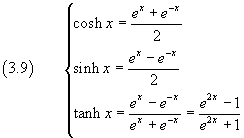## 3. The natural exponential function

If we sum term by term the addition formulas (2.6) we get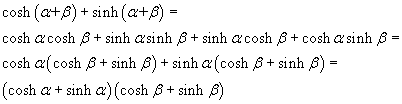therefore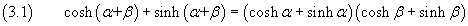If we call exp(x) the sum of the hyperbolic cosine and sine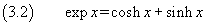from (3.1) we have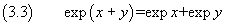From (3.3) we can deduce that the function exp(x) is a power, because it has a typical property of the powers: the product of two powers of the same base is a power of the same base in which the exponent is the sum of the multiplied powers exponents.

The function exp(x) is said natural exponential and its base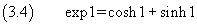is usually written e and, sometimes, said the Napier's constant.

The number e is a transcendental irrational whose value, which can be approximated in many ways, is about 2.71828182.

So we can express the function exp(x) as a power of e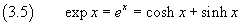cosh x and sinh x are defined over ℜ, so is their sum ex.

cosh x is always positive and its absolute value is always greater than that of sinh x, so ex is always positive.

If x>0, ex>1, so ex always increases. In fact, for each positive h,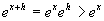Since ex always increases, it has neither maximum nor minimum.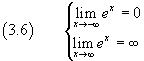Since the derivative of a sum is the sum of the derivatives of its addends, we have also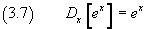From (3.5) we have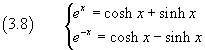and immediately